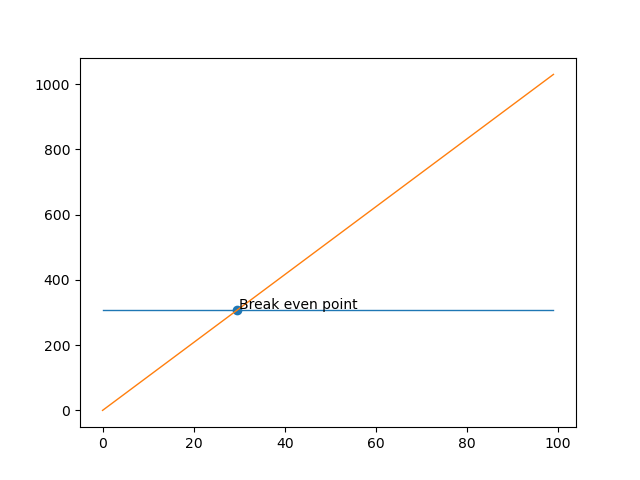## Thursday, June 25, 2020

### How much do I have to walk to burn off my Guinness calories?

﻿ Welcome file
This was a real question I had. Fortunately, its pretty simple algebra and I know how to do that so lets start with gathering a couple of numbers.
1. How many calories do I burn per minute by walking? 7.7 I walk quite vigorously
• $230/30=7.7$ Calories per minute
2. How many calories do I take in per ounce of Guinness? 10.4 It might be 10.5 but this is what I got
• $125/12=10.4$ Calories per ounce
Now we know every minute I walk burns 7.7 calories and every ounce of Guinness I drink gains me 10.4 calories. So the next question is how many minutes do I have to walk to burn off 1 ounce of Guinness. More math.
• $10.4/7.7=1.35$ minutes (1 minute 21 seconds) walked to burn 1 ounce of Guinness Calories
So the closest bar to me (shouts to DD Peckers) is ~20 mins in one direction. How many Guinness can I have to break even by walking there and back. (Its unfortunately not very many)
Given a Guinness is 20 oz (imperial pint) and my walk to and from the bar is ~40 mins:
• $40*7.7/10.4/20=1.48$ Guinness
Visualized as:
%matplotlib notebook
import matplotlib.pyplot as plt
plt.plot([[7.7*40] for x in range(100)], linewidth=1, label='random')
plt.plot([[x*10.4] for x in range(100)], linewidth=1, label='random')
plt.scatter([7.7*40/10.4], [7.7*40])
plt.annotate("Break even point", (30, 310))
plt.show()The orange line in this case is the increase of calories per ounce drank and the blue line is a constant of calories burned if I walk vigorously for 40 mins. The intersection gives us the amount of ounces it takes to equal the number of calories I burned walking 40 mins. Which shows x as 29.6 ounces divided by 20 is 1.48 Guinness.

I wish my story problems as a kid were as interesting as this, but alas they were not, so here we are making up my own.